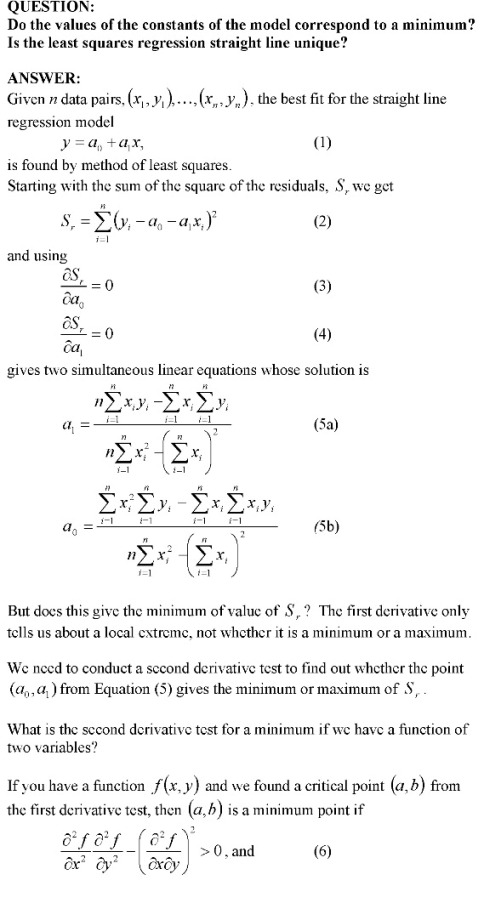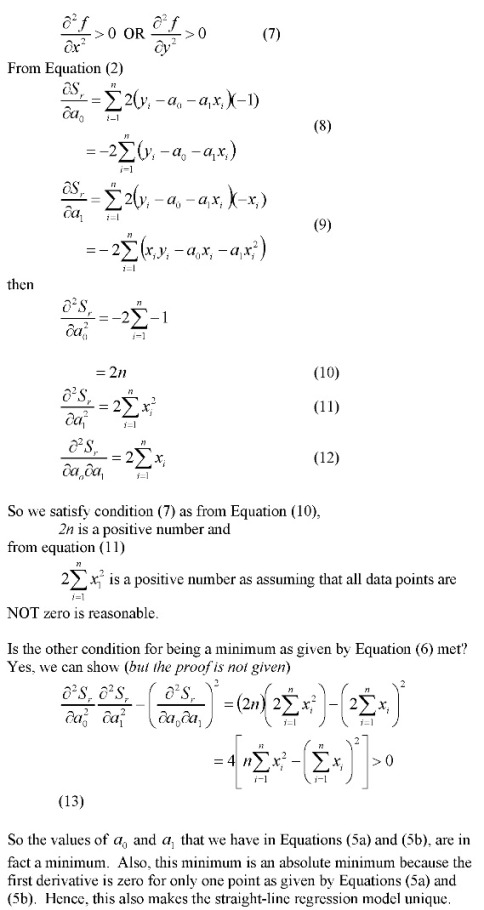# How do you know that the least squares regression line is unique and corresponds to a minimum

We already know that using the criterion of either

1. minimizing sum of residuals OR
2. minimizing sum of the absolute value of residuals

is BAD as either of the criteria do not give a unique line. Visit these notes for an example where these criteria are shown to be inadequate.

So we use minimizing the sum of the squares of the residuals as the criterion. How can we show that this criterion gives a unique line?

The proof is given below as image files because the proof is equation intensive. I made a better resolution pdf file also._____________________________________________________

This post is brought to you by Holistic Numerical Methods: Numerical Methods for the STEM undergraduate at http://nm.mathforcollege.com

## 0 thoughts on “How do you know that the least squares regression line is unique and corresponds to a minimum”

1.Jason McCarty says:

Nice. Note that (13) is positive because it’s a multiple of the variance of the x_i’s. Of course, if all the x_i’s are equal, the variance is zero, so having the x_i’s not all equal is necessary and sufficient for uniqueness.

But a single critical point which is a local minimum need not be a global minimum, see http://en.wikipedia.org/wiki/Maxima_and_minima . Here I think you’re OK since the Hessian is positive everywhere.

2.Jason McCarty says:

Nice. Note that (13) is positive because it’s a multiple of the variance of the x_i’s. Of course, if all the x_i’s are equal, the variance is zero, so having the x_i’s not all equal is necessary and sufficient for uniqueness.

But a single critical point which is a local minimum need not be a global minimum, see http://en.wikipedia.org/wiki/Maxima_and_minima . Here I think you’re OK since the Hessian is positive everywhere.